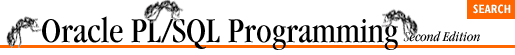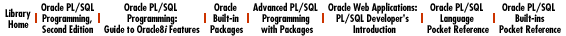home | O'Reilly's CD bookshelfs | FreeBSD | Linux | Cisco | Cisco Exam

### C.11 DBMS_ROWID (PL/SQL8 Only)

Use the DBMS_ROWID package to work with ROWIDs from within PL/SQL programs and SQL statements. Remember that as of Oracle8, there are two types of ROWIDs: extended and restricted. Restricted ROWIDs are the ROWIDs available with Oracle Version 7 and earlier. Extended ROWIDs are used in Oracle8.

### C.11.1 The ROWID_CREATE function

Call the create function to create a ROWID (either restricted or extended as you request) based on the individual ROWID component values you specify. This should be used for test purposes only. The specification is:

```FUNCTION DBMS_ROWID.ROWID_CREATE
(rowid_type IN NUMBER,
object_number IN NUMBER,
relative_fno IN NUMBER,
block_number IN NUMBER,
row_number IN NUMBER)
RETURN ROWID;```

### C.11.2 The ROWID_INFO procedure

The info procedure returns information about the specified ROWID. This procedure essentially "parses" the ROWID. The specification is:

```PROCEDURE DBMS_ROWID.ROWID_INFO
(rowid_in IN ROWID,
rowid_type OUT NUMBER,
object_number OUT NUMBER,
relative_fno OUT NUMBER,
block_number OUT NUMBER,
row_number OUT NUMBER);```

### C.11.3 The ROWID_TYPE function

The type function determines if the ROWID is restricted or extended. It returns if the ROWID is restricted, and 1 if the ROWID is extended. The specification is:

`FUNCTION DBMS_ROWID.ROWID_TYPE (row_id IN ROWID) RETURN NUMBER;`

### C.11.4 The ROWID_OBJECT function

Use the object function to return the data object number for an extended ROWID. The object function returns if the specified ROWID is restricted. The specification is:

`FUNCTION DBMS_ROWID.ROWID_OBJECT (row_id IN ROWID) RETURN NUMBER;`

### C.11.5 The ROWID_RELATIVE_FNO function

The relative_fno function returns the relative file number (relative to the tablespace) of the ROWID. The specification is:

`FUNCTION DBMS_ROWID.ROWID_RELATIVE_FNO (row_id IN ROWID) RETURN NUMBER;`

### C.11.6 The ROWID_BLOCK_NUMBER function

Use the block_number function to return the database block number of the ROWID. The specification is:

`FUNCTION DBMS_ROWID.ROWID_BLOCK_NUMBER (row_id IN ROWID) RETURN NUMBER;`

### C.11.7 The ROWID_ROW_NUMBER function

The row_number function returns the row number of the ROWID. The specification is:

`FUNCTION DBMS_ROWID.ROWID_ROW_NUMBER (row_id IN ROWID) RETURN NUMBER;`

### C.11.8 The ROWID_TO_ABSOLUTE_FNO function

Call the to_absolute_fno function to return the absolute file number (for a row in a given schema and table) from the ROWID. The specification is:

```FUNCTION DBMS_ROWID.ROWID_TO_ABSOLUTE_FNO
(row_id IN ROWID,
schema_name IN VARCHAR2,
object_name IN VARCHAR2)
RETURN NUMBER;```

### C.11.9 The ROWID_TO_EXTENDED function

The to_extended function converts a restricted ROWID to an extended ROWID. The specification is:

```FUNCTION DBMS_ROWID.ROWID_TO_EXTENDED
(old_rowid IN ROWID,
schema_name IN VARCHAR2,
object_name IN VARCHAR2,
conversion_type IN INTEGER)
RETURN ROWID;```

### C.11.10 The ROWID_TO_RESTRICTED function

The to_restricted function converts an extended ROWID to a restricted ROWID. The specification is:

```FUNCTION DBMS_ROWID.ROWID_TO_RESTRICTED
(old_rowid IN ROWID,
conversion_type IN INTEGER)
RETURN ROWID;```

### C.11.11 The ROWID_VERIFY function

Use the verify function to determine whether a restricted ROWID can be converted to an extended format. The verify function returns if the ROWID provided can be converted and 1 otherwise. The specification is:

```FUNCTION DBMS_ROWID.ROWID_VERIFY
(rowid_in IN ROWID,
schema_name IN VARCHAR2,
object_name IN VARCHAR2,
conversion_type IN INTEGER)
RETURN NUMBER;

```